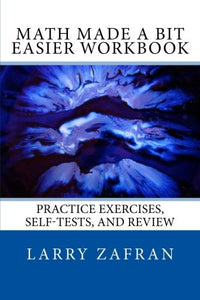# Math Made A Bit Easier Workbook: Practice Exercises, Self-Tests, And Review

• Publish Date: 2009-12-11
• Binding: Paperback
• Author: Larry Zafran
Regular price \$29.76 Sale price \$14.16

Attention: For textbook, access codes and supplements are not guaranteed with used items.

This is the second book in the Math Made a Bit Easier series by independent math tutor Larry Zafran. It is a workbook of practice exercises, self-tests, and review notes to be used in conjunction with the first book in the series, subtitled Basic Math Explained in Plain English.

The math content in this book is directly aligned with the first book. It covers the topics which comprise the foundation of math. It begins with practice in basic arithmetic, followed by basic operations, negative numbers, fractions, decimals, percents, and basic probability and statistics. If these topics are not completely mastered, later work will prove to be quite difficult. This is especially true of algebra.

An extensive introduction describes how to obtain the greatest benefit from the book. The book also outlines practical techniques for attaining the optimal mindset for studying math and improving scores on exams. An answer key for all exercises and self-tests is included.